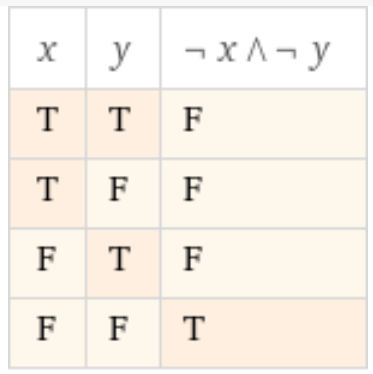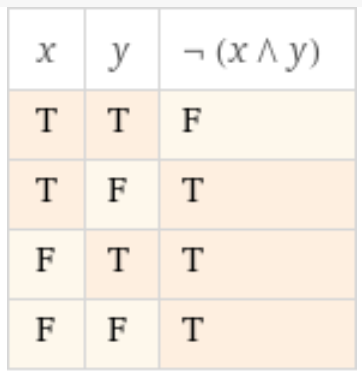# While...wend and condition

I try to do a ‘while…wend’ until two variables are 127, but it quits if just one variable is 127.
What is wrong here?

``````while (data1 <> 127 and data2 <> 127)
get data1
get data2
//do something
wend
``````

No idea, but have-you tried the other way of testing the values ?

``````While
get data1
get data2
//do something
Wend (data1 = 127 And data2 = 127)
``````
``````While ( Not (data1 = 127 or data2 = 127) )
``````

The equivalent using “And” should be:

``````While ( Not(data1 = 127) And Not(data2 = 127) )

//So it ends equivalent to your statement

While (data1 <> 127 and data2 <> 127)
``````

I guess you have some problem with your data.

(Edit: I was misunderstanding his problem at this point)

I understand he want to stop when both values are 127.

Was I wrong (time for an afternoon sleep ?)?

I understood he wants to quit if one is 127

So I need more coffee

If only I could send you one…I’m old and these days I feel like I do not understand correctly things, so I asked.

However, this does not disallow me for an afternoon nap !

For a continue until both = 127 is

``````While ( Not(data1 = 127 And data2 = 127) )
``````

Loop … Until both = 127…

You could also exit the while``````while true
// Do super secret stuff

if data1 = 127 and data2 = 127 then
// Time to leave!
exit while

end if

wend
``````
1 Like

The design he proposes seems that he does not want to enter the loop if the condition is previously set, so I stick with:

Or

``````Do Until data1 = 127 And data2 = 127
//...
Loop
``````
1 Like

OK.

OK. alone is rejected. Error is:
`Body seems unclear, is it a complete sentence?`

Correct, I want a quit if both are 127.

It’s OK, I also found a workaround, but I just want to understand why the and condition not works…

^^^

2 Likes

Your first expression was wrong for what you intended.

You wrote the equivalent to:But you wanted1 Like

While loopes while the test is true. If you write:
`while (data1 <> 127 and data2 <> 127)`
If data1 and data2 are differents, then it is true and true then it loops.
If one is egual it is true and false (or false and true) = false then it exists.
By the way, I always write:
`while (data1 <> 127) and (data2 <> 127)`
with () for each test. I’m always afraid of the interpretation of the compiler.

2 Likes

The compiler don’t make assumptions, it just executes what you wrote. Be afraid of what you wrote.2 Likes

it quits if just one variable is 127.
What is wrong here?

When dealing with files, we are told to test in this order:

`if thefile <> nil and thefile.exists then`

if the first test is false, the compiler doesnt bother testing the second.

So

data1 <> 127 is FALSE because it IS 127
therefore the compiler feels no need to check whether data2 <> 127

The test should be simply test that both are 127 as Rick says

so
`While ( Not(data1 = 127) And Not(data2 = 127) )`

2 Likes

Thank you guys, now I understand.

1 Like

I would find:

``````While  ((data1=127 and data2=127) = False)
...
Wend
``````